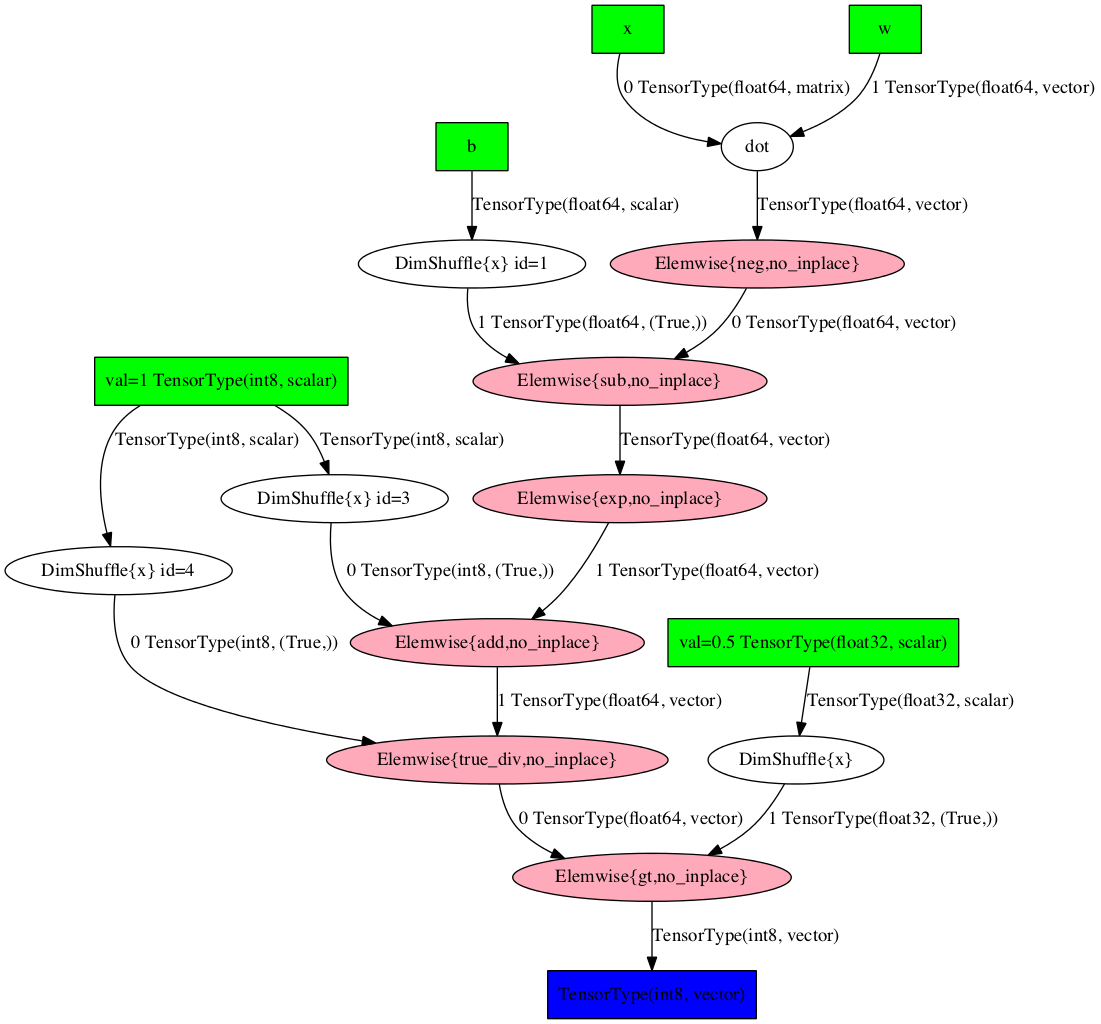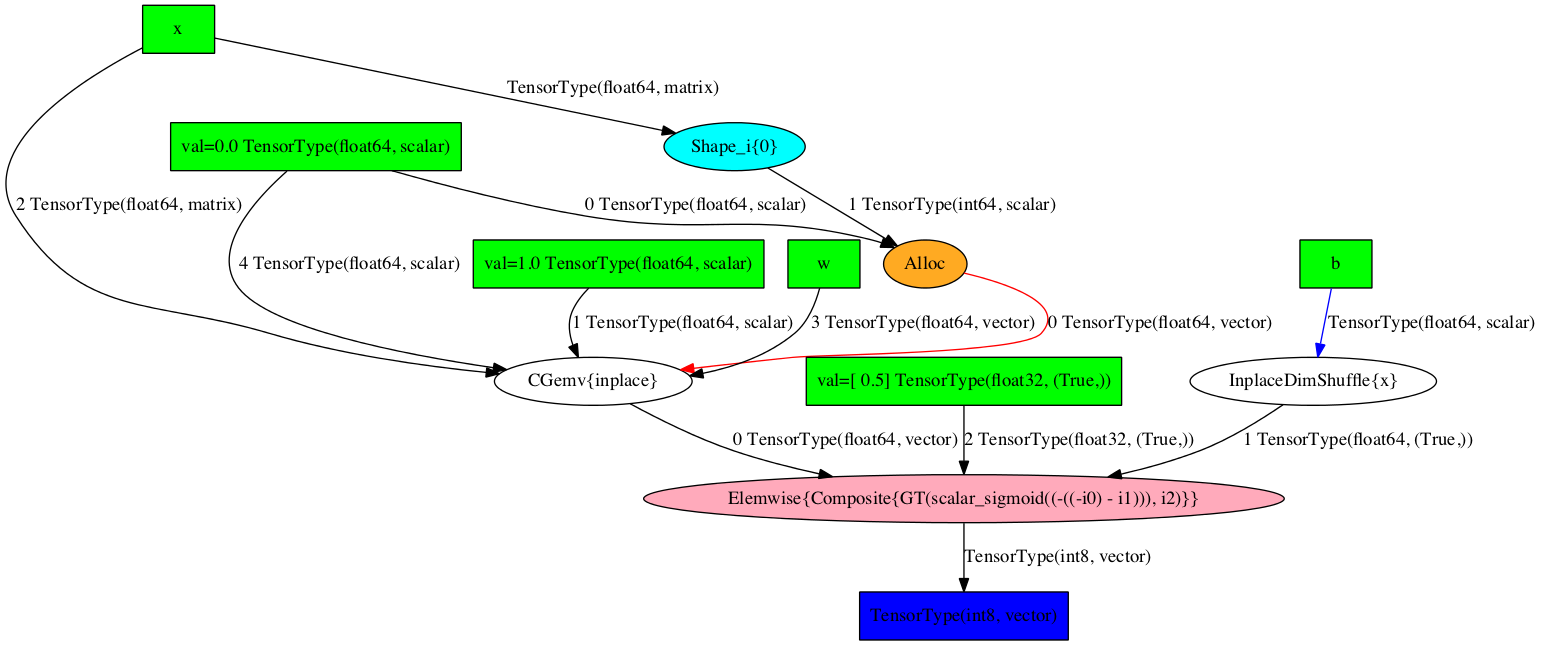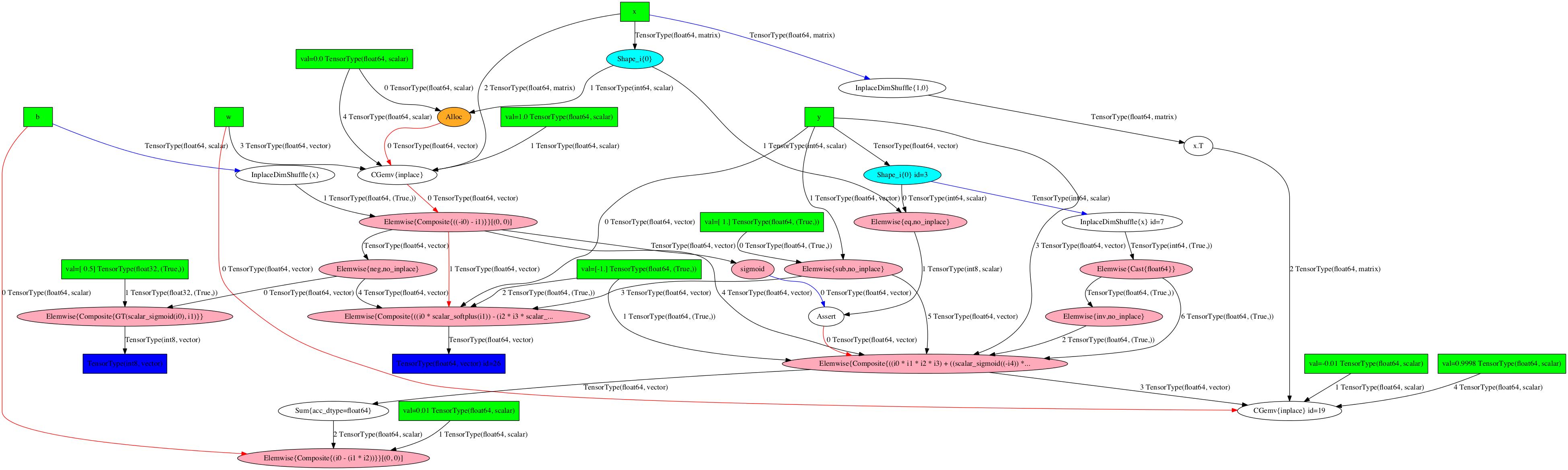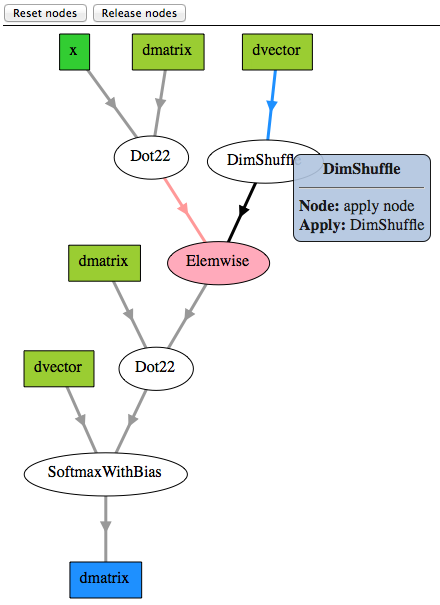# Printing/Drawing PyTensor graphs#

PyTensor provides the functions pytensor.printing.pprint() and pytensor.printing.debugprint() to print a graph to the terminal before or after compilation. pprint() is more compact and math-like, debugprint() is more verbose. PyTensor also provides pydotprint() that creates an image of the function. You can read about them in printing – Graph Printing and Symbolic Print Statement.

Note

When printing PyTensor functions, they can sometimes be hard to read. To help with this, you can disable some PyTensor rewrites by using the PyTensor flag: optimizer_excluding=fusion:inplace. Do not use this during real job execution, as this will make the graph slower and use more memory.

Consider again the logistic regression example:

>>> import numpy as np
>>> import pytensor
>>> import pytensor.tensor as at
>>> rng = np.random.default_rng(2382)
>>> # Training data
>>> N = 400
>>> feats = 784
>>> D = (rng.standard_normal(N, feats).astype(pytensor.config.floatX), rng.integers(size=N,low=0, high=2).astype(pytensor.config.floatX))
>>> training_steps = 10000
>>> # Declare PyTensor symbolic variables
>>> x = at.matrix("x")
>>> y = at.vector("y")
>>> w = pytensor.shared(rng.standard_normal(feats).astype(pytensor.config.floatX), name="w")
>>> b = pytensor.shared(np.asarray(0., dtype=pytensor.config.floatX), name="b")
>>> x.tag.test_value = D
>>> y.tag.test_value = D
>>> # Construct PyTensor expression graph
>>> p_1 = 1 / (1 + at.exp(-at.dot(x, w)-b)) # Probability of having a one
>>> prediction = p_1 > 0.5 # The prediction that is done: 0 or 1
>>> xent = -y*at.log(p_1) - (1-y)*at.log(1-p_1) # Cross-entropy
>>> cost = xent.mean() + 0.01*(w**2).sum() # The cost to optimize
>>> # Training and prediction function
>>> train = pytensor.function(inputs=[x,y], outputs=[prediction, xent], updates=[[w, w-0.01*gw], [b, b-0.01*gb]], name = "train")
>>> predict = pytensor.function(inputs=[x], outputs=prediction, name = "predict")


## Pretty Printing#

>>> pytensor.printing.pprint(prediction)
'gt((TensorConstant{1} / (TensorConstant{1} + exp(((-(x \\dot w)) - b)))),
TensorConstant{0.5})'


## Debug Print#

The pre-compilation graph:

>>> pytensor.printing.debugprint(prediction)
Elemwise{gt,no_inplace} [id A] ''
|Elemwise{true_div,no_inplace} [id B] ''
| |InplaceDimShuffle{x} [id C] ''
| | |TensorConstant{1} [id D]
|   |InplaceDimShuffle{x} [id F] ''
|   | |TensorConstant{1} [id D]
|   |Elemwise{exp,no_inplace} [id G] ''
|     |Elemwise{sub,no_inplace} [id H] ''
|       |Elemwise{neg,no_inplace} [id I] ''
|       | |dot [id J] ''
|       |   |x [id K]
|       |   |w [id L]
|       |InplaceDimShuffle{x} [id M] ''
|         |b [id N]
|InplaceDimShuffle{x} [id O] ''
|TensorConstant{0.5} [id P]


The post-compilation graph:

>>> pytensor.printing.debugprint(predict)
Elemwise{Composite{GT(scalar_sigmoid((-((-i0) - i1))), i2)}} [id A] ''   4
|...Gemv{inplace} [id B] ''   3
| |AllocEmpty{dtype='float64'} [id C] ''   2
| | |Shape_i{0} [id D] ''   1
| |   |x [id E]
| |TensorConstant{1.0} [id F]
| |x [id E]
| |w [id G]
| |TensorConstant{0.0} [id H]
|InplaceDimShuffle{x} [id I] ''   0
| |b [id J]
|TensorConstant{(1,) of 0.5} [id K]


## Picture Printing of Graphs#

The pre-compilation graph:

>>> pytensor.printing.pydotprint(prediction, outfile="pics/logreg_pydotprint_prediction.png", var_with_name_simple=True)
The output file is available at pics/logreg_pydotprint_prediction.pngThe post-compilation graph:

>>> pytensor.printing.pydotprint(predict, outfile="pics/logreg_pydotprint_predict.png", var_with_name_simple=True)
The output file is available at pics/logreg_pydotprint_predict.pngThe optimized training graph:

>>> pytensor.printing.pydotprint(train, outfile="pics/logreg_pydotprint_train.png", var_with_name_simple=True)
The output file is available at pics/logreg_pydotprint_train.png## Interactive Graph Visualization#

The new d3viz module complements pytensor.printing.pydotprint() to visualize complex graph structures. Instead of creating a static image, it generates an HTML file, which allows to dynamically inspect graph structures in a web browser. Features include zooming, drag-and-drop, editing node labels, or coloring nodes by their compute time.

=> d3viz <=